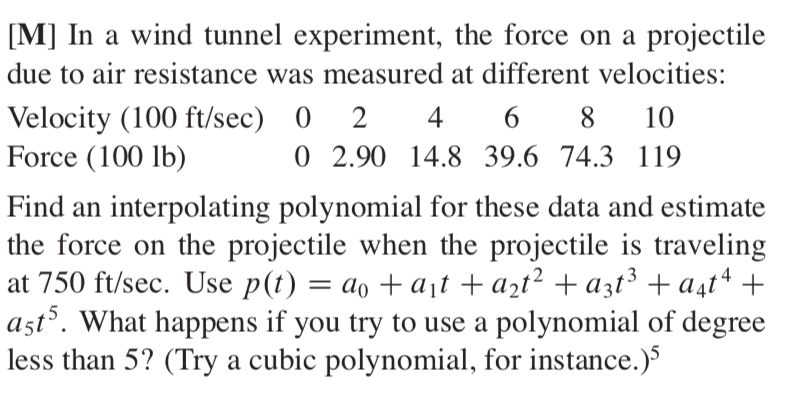# Deciphering this problem statement: Air resistance on projectiles

Homework Statement:
See attached image
Relevant Equations:
basic augmented matriciesI am struggling with our equation and where to plug in my velocities and forces? After looking at my book I don't understand why p(t) would be where I plug in force, and why variable "t" is where we plug our velocity in?

When the problem says "what happens if you try to use a polynomial of degree less than 5" do they mean have an equation like:
p(t)=a0+a1t+a2t^2+a3t^3 ?

Any help would be appreciated.
Thanks

Homework Helper
Gold Member
2022 Award
Homework Statement:: See attached image
Homework Equations:: basic augmented matricies

I don't understand why p(t) would be where I plug in force, and why variable "t" is where we plug our velocity in?
p is a function, not a variable. ##p(t)=a0+a1t+a2t^2+a3t^3## says that if you supply the input value t to the function p it will return the value ##a0+a1t+a2t^2+a3t^3##. t just stands for any arbitrary input you might wish to make.
You want to express a force, F, as a polynomial function of wind speed, w. That means you are looking for a polynomial p such that ##F=p(w)##. So in p(t) the input p is to take the values you have for w, and the polynomial is to return something close to the observed values of F: ##F_i\approx p(w_i)##

•archaic
Homework Statement:: See attached image
Homework Equations:: basic augmented matricies

When the problem says "what happens if you try to use a polynomial of degree less than 5" do they mean have an equation like:
p(t)=a0+a1t+a2t^2+a3t^3 ?
Yes.
By now, I think you have figured that you must find those constants in that polynomial. How do you think you can do that? Look at what you have been given.

Homework Helper
There is a crank-and-grind approach to constructing an interpolating polynomial through a given set of data points. The Wiki page gives it, introduced by the words: "Alternatively, we may write down the polynomial immediately in terms of Lagrange polynomials:"

The idea behind that construction is that one can easily write down a polynomial which is zero at all but one of the given data points. For instance, a polynomial which is zero at every given data point other than t=4 is:$$(t-0)(t-2)(t-6)(t-8)(t-10)$$ You want to scale that polynomial up or down so that its result, when evaluated at t=4 is equal to the given data value for t=4. i.e. it needs to evaluate to 14.8. You determine the required scaling factor by taking the given data value (14.8) and dividing it by the result of evaluating the polynomial at t=4.

i.e. you evaluate:$$\frac{(t-0)(t-2)(t-6)(t-8)(t-10)}{(4-0)(4-2)(4-6)(4-8)(4-10)}14.8$$ as a polynomial. That is, it should come out as$$\text{something }t^5 + \text{something }t^4 + \text{something }t^3 + \text{something }t^2 + \text{something }t + \text{something}$$ You then repeat for each of the other data points and add all six polynomials together. Because of the way they were constructed, each individual formula is a fifth degree polynomial, is zero at all but one point and takes on the correct value at that data point. The sum of all of them must therefore be a fifth (or fewer) degree polynomial that takes the correct value at all the data points.

It is a fair bit of mind-numbing work.

Edit: Switched to use "t" as the dummy variable in the polynomial as the question suggests.

Last edited:
Gold Member
The manual process suggested by jbriggs444 will certainly work.

For something less painful, you might consider using a spreadsheet such as MS Excel, and looking up the "generalized least square" method.

A word of caution regarding the use of Excel: you can ask it for a 5th degree polynomial trendline and "display equation on Chart" but the default will display only 2 decimal places for the coefficients. For a 5th degree polynomial that is just not enough to use it to get good values. If you go the trendline route, you need to format the trendline equation to show 5 or 6 figures.

Gold Member
A word of caution regarding the use of Excel: you can ask it for a 5th degree polynomial trendline and "display equation on Chart" but the default will display only 2 decimal places for the coefficients. For a 5th degree polynomial that is just not enough to use it to get good values. If you go the trendline route, you need to format the trendline equation to show 5 or 6 figures.

I was not talking about using the trend line function. Though, of course, that's possible. The digits displayed can be formatted to show the desired number of digits.

I was talking about using the spreadsheet to do the generalized least square fit to a polynomial of your choice. You make a column with all 1's, a column with x, a column with x-squared, a column with x-cubed, etc. Then you build your polynomial out of A times the first column, B times the second, etc. Then you minimize the sum of the squares of the differences with your data by adjusting the parameters. You get the same notion as a least-square fit to a straight line, but to a polynomial. There's a keen little matrix equation that you need to solve to get the minimum. And such matrix equations, up to a few 100 data points and up to a dozen columns, is easily within Excel's ability.

Excel also has a built-in polynomial fitting function. This will work directly on the spreadsheet, no graph required.

I know you weren't.